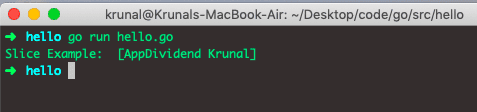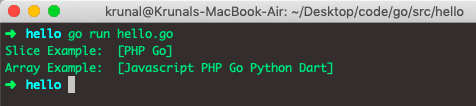# Golang Slice: The Complete Guide

Golang array has a fixed size but Go slice, on the other hand, is a dynamically-sized, flexible view into the elements of an array. To install Golang on MacOS, check out my How To Install Go On Mac tutorial.

## Golang Slice

Golang slice is a dynamically-sized, flexible view array. Go slice is a reference to a contiguous segment of an array. A pointer with additional properties about the array. Let me clarify: Golang Slice and Golang Array are different data types.

A Slice is the segment of an array. The slices build on arrays and provide more power, flexibility, and convenience compared to the arrays.

Unlike the arrays, slices are typed only by the elements they contain.

Slices support several more that make them richer than arrays. One of the built-in functions is the slice append(), which returns a slice containing one or more new values.

Let’s see the syntax of Slices in Golang.

## How to define a slice in Golang

To define a slice in golang, use the var variablename []data type syntax.

`var variablename []data type`

Let’s define an actual slice.

`var sl = []string`

Let’s declare a slice.

`var sl = []string{"AppDividend", "Krunal"}`

Now let’s take an example and print the slice on the console.

```/* hello.go */

package main

import "fmt"

func main() {
var sl = []string{"AppDividend", "Krunal"}

fmt.Println("Slice Example: ", sl)
}
```

So, in the main() function, we have defined the slice using two strings and then displayed the slice on the console. Run the file using the following command.

`go run hello.go`

The output is the following.We can also initialize the slice like the following code.

```/* hello.go */

package main

import "fmt"

func main() {
sl := []string{
"AppDividend",
"Krunal"}

fmt.Println("Slice Example: ", sl)
}
```

We will get the same output.

## Golang slice vs array

The main difference between slice and array is that slices are indexable and have a length. But unlike arrays, they can be resized. In terms of programming paradigm, The slice is just a reference to the underlying array.

## How to create a slice from an array

Since the slice is the segment of an array, we can create the slice from an array.

Let’s take the following example.

```/* hello.go */

package main

import "fmt"

func main() {
var a = string{"Javascript", "PHP", "Go", "Python", "Dart"}
var b = a[1:3]
fmt.Println("Slice Example: ", b)
fmt.Println("Array Example: ", a)
}
```

Here, first, we have defined a fixed-length array called a.

Since the slice is formed by specifying two indices, a low and high bound, separated by a colon.

`a[1:3]`

That means, from an array a, start the element from 0 and pick the values between 1(low) to 3(high). Which means we will form the slice whose index in an array is and 2.

In the above example, PHP and Go have index 1 and 2. So the output slice will be of those elements.

Let’s see the output of Slice and Array in the console.The low and high indices in a slice expression are optional. The default value for low is 0, and high is the length of a slice.

A slice is like an array which is a container to hold elements of the same data type, but a slice can vary in size.

That’s it for this tutorial.

## Recommended Posts

Golang slice append

Golang for loop

Golang custom-type declaration